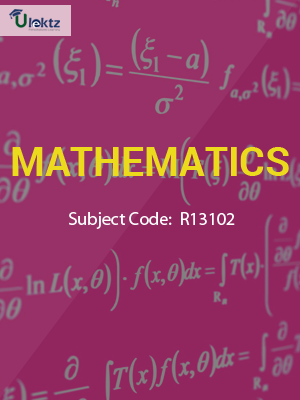•My WalletMy Order
•My Profile
•My Connections
•My Books
•My Videos
•My Tests
•My Calender
•My Messages
•My Shopping Cart
•My Orders
•Account Settings
•Help

# Book Details# Mathematics-I

 Course Code : R13102 Author : uLektz University : JNTU Kakinada Regulation : 2016 Categories : General Engineering Format :ePUB3 (DRM Protected) Type : eBook

FREE

Description :Mathematics-I of R13102 covers the latest syllabus prescribed by JNTU Kakinada for regulation 2016. Author: uLektz, Published by uLektz Learning Solutions Private Limited.

Note : No printed book. Only ebook. Access eBook using uLektz apps for Android, iOS and Windows Desktop PC.

##### Topics
###### UNIT I: Differential equations of first order and first degree:

1.1 Linear equation

1.2 Bernoulli equation

1.3 Exact-Reducible to exact.

1.4 Applications : Newton’s Law of cooling-Law of natural growth and decay orthogonal trajectories.

###### UNIT II: Linear differential equations of higher order:

2.1 Non-homogeneous equations of higher order with constant coefficients with RHS term of the type e ax, Sin ax, cos ax, polynomials in x, e axV(x), xV(x).

2.2 Applications : LCR circuit, Simple Harmonic motion

###### UNIT III Laplace transforms:

3.1 Laplace transforms of standard functions

3.2 Shifting Theorems, Transforms of derivatives and integrals

3.3 Unit step function –Dirac’s delta function

3.4 Inverse Laplace transforms

3.5 Convolution theorem (with out proof).

3.6 Application : Solutions of ordinary differential equations using Laplace Transforms.

###### UNIT IV Partial differentiation:

4.1 Introduction

4.2 Total derivative-Chain rule

4.3 Generalized Mean Value theorem for single variable (without proof)

4.4 Taylors and Mc Laurent’s series for two variables– Functional dependence

4.5 Jacobian.

4.6 Applications: Maxima and Minima of functions of two variables with constraints and without constraints.

###### UNIT V First order Partial differential equations:

5.1 Formation of partial differential equations by elimination of arbitrary constants and arbitrary functions

5.2 Solutions of first order linear (Lagrange) equation and nonlinear (standard type) equations

###### UNIT VI Higher order Partial differential equations:

6.1 Solutions of Linear Partial differential equations with constant coefficients

6.2 Method of separation of Variables

6.3 Applications : One- dimensional Wave, Heat equations - two-dimensional Laplace Equation.

### Related Books